### Sensitivity Analysis

Sensitivity analysis is a technique for examining the effects of changes in model parameters on the optimal solution. The analysis enables you to examine the size of a perturbation to the right-hand-side or objective vector by an arbitrary change vector for which the basis of the current optimal solution remains optimal.

Note: When sensitivity analysis is performed on integer-constrained problems, the integer variables are fixed at the value they obtained in the integer optimal solution. Therefore, care must be used when interpreting the results of such analyses. Care must also be taken when preprocessing is enabled, because preprocessing usually alters the original formulation.

#### Right-Hand-Side Sensitivity Analysis

Consider the problem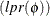: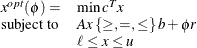whereis a right-hand-side change vector.

Let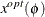denote an optimal basic feasible solution to. PROC LP can be used to examine the effects of changes inon the solution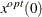of problem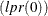. For the basic solution, let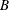be the matrix composed of the basic columns of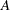and let
be the matrix composed of the nonbasic columns of. For the basis matrix, the basic components of, written as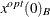, can be expressed as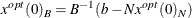Furthermore, becauseis feasible,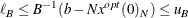where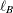is a column vector of the lower bounds on the structural basic variables, and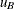is a column vector of the upper bounds on the structural basic variables. For each right-hand-side change vectoridentified in the RHSSEN statement, PROC LP finds an interval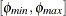such that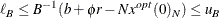for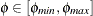. Furthermore, because changes in the right-hand side do not affect the reduced costs, for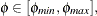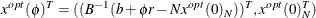is optimal in.

For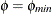and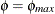, PROC LP reports the following:

• the names of the leaving variables

• the value of the optimal objective in the modified problems

• the RHS values in the modified problems

• the solution status, reduced costs and activities in the modified problems

The leaving variable identifies the basic variablethat first reaches either the lower bound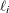or the upper bound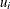asreaches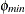or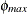. This is the basic variable that would leave the basis to maintain primal feasibility. Multiple RHSSEN variables can appear in a problem data set.

#### Price Sensitivity Analysis

Consider the problem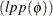: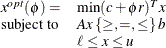whereis a price change vector.

Letdenote an optimal basic feasible solution to. PROC LP can be used to examine the effects of changes inon the solutionof problem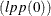. For the basic solution, letbe the matrix composed of the basic columns ofand letbe the matrix composed of the nonbasic columns of. For basis matrix, the reduced cost associated with theth variable can be written as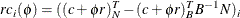where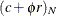and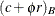is a partition of the vector of price coefficients into nonbasic and basic components. Becauseis optimal in, the reduced costs satisfy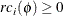if the nonbasic variable in columnis at its lower bound, and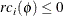if the nonbasic variable in columnis at its upper bound.

For each price coefficient change vectoridentified with the keyword PRICESEN in the TYPE variable, PROC LP finds an intervalsuch that for,if the nonbasic variable in columnis at its lower bound, andif the nonbasic variable in columnis at its upper bound. Because changes in the price coefficients do not affect feasibility, for,is optimal in. Forand, PROC LP reports the following:

• the names of entering variables

• the value of the optimal objective in the modified problems

• the price coefficients in the modified problems

• the solution status, reduced costs, and activities in the modified problems

The entering variable identifies the variable whose reduced cost first goes to zero asreachesor. This is the nonbasic variable that would enter the basis to maintain optimality (dual feasibility). Multiple PRICESEN variables may appear in a problem data set.